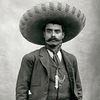## Welcome to the Treehouse Community

Want to collaborate on code errors? Have bugs you need feedback on? Looking for an extra set of eyes on your latest project? Get support with fellow developers, designers, and programmers of all backgrounds and skill levels here with the Treehouse Community!

### Looking to learn something new?

Treehouse offers a seven day free trial for new students. Get access to thousands of hours of content and join thousands of Treehouse students and alumni in the community today.# JS error. Cannot read property 'text' of undefined...unsure what

Hello,

I was doing an exercise for the Object-Oriented JS course with Chalkey and I have come to a standstill. I run my code and in the console, I get an error of "uncaught TypeError: Cannot read property 'text' of undefined. Any help is appreciated.

```var QuizUI = {
displayNext: function () {
if (quiz.hasEnded()) {  //checks if the quiz has ended
this.displayScore();  //displays score
} else {
this.displayQuestion(); // gets the text from the current question and inserts into #question
this.displayChoices();  //displays what question we are currently on
this.displayProgress();
}
},
displayQuestion: function() {
this.populateIdWithHTML("question", quiz.getCurrentQuestion().text); //this is where the error is somewhere...
},
displayChoices: function() {
var choices = Quiz.getCurrentQuestion().choices;

for (var i = 0; i < choices.length; i++) {
this.populateIdWithHTML("Choice" + choices[i]);
this.guessHandler("guess" + i, choices[i]);
}
},
displayScore: function() {
var gameOverHTML = '<h1>Game Over</h1>';
gameOverHTML += "<h2> Your score is: " + quiz.score + "</h2>";
this.populateIdWithHTML( "quiz", gameOverHTML );
},

populateIdWithHTML: function(id, text) {
var element = Document.getElementById(id);
element.innerHTML = text;
},

guessHandler : function(id, guess) {
var button = document.getElementById(id);
button.onclick = function() {
quiz.guess(guess);
QuizUI.displayNext();
}
},

displayProgress: function() {
var currentQuestionNumber = quiz.currentQuizIndex + 1;
this.populateIdWithHTML("progress","Question " + currentQuestionNumber + " of " + quiz.questions.length);
},

}
```The error means that quiz.getCurrentQuestion() returns undefined, so take a look at your implementation of that method. If you cannot see the problem in quiz.getCurrentQuestion(), then you can post the code for quiz, and I will help by explaining exactly what is wrong with quiz.getCurrentQuestion().Hello Benjamin,

Here is my code:

quiz.js

```function Quiz(questions) {
this.score = 0;
this.questions = questions;
this.currentQuestionIndex = 0;
}

this.score++;
}
this.currentQuestionIndex++;
};

Quiz.prototype.getCurrentQuestion = function() {
return this.questions[this.currentQuestionsIndex];
};

Quiz.prototype.hasEnded = function() {
return this.currentQuestionIndex >= this.questions.length;
};
```

question.js

```function Question (text, choices, answer) {
this.text = text;
this.choices = choices;
}

};
```

app.js

```//Create questions
var questions = [
new Question("Who is the best?",["Hector", "Sancho"], "Sancho"),
new Question("Who is the worst?",["Hector", "Sancho"], "Sancho")
];

//Create quiz
var quiz = new Quiz (questions);

//Display quiz
QuizUI.displayNext();
```There is a typo in the following code in quiz.js:

```Quiz.prototype.getCurrentQuestion = function() {
return this.questions[this.currentQuestionsIndex];
};
```

this.currentQuestionsIndex should be this.currentQuestionIndex.